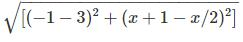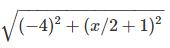Courses

# Test: Numeric Entry- 1

## 15 Questions MCQ Test Section-wise Tests for GRE | Test: Numeric Entry- 1

Description
This mock test of Test: Numeric Entry- 1 for GRE helps you for every GRE entrance exam. This contains 15 Multiple Choice Questions for GRE Test: Numeric Entry- 1 (mcq) to study with solutions a complete question bank. The solved questions answers in this Test: Numeric Entry- 1 quiz give you a good mix of easy questions and tough questions. GRE students definitely take this Test: Numeric Entry- 1 exercise for a better result in the exam. You can find other Test: Numeric Entry- 1 extra questions, long questions & short questions for GRE on EduRev as well by searching above.
*Answer can only contain numeric values
QUESTION: 1

### The denominator of a fraction is 4 less than the numerator. If the denominator is decreased by 2 and the numerator is increased by 1, then the numerator is eight times the denominator. Find the numerator of the fraction.

Solution:

Let the fraction be x/y
According to the conditions y = x - 4 x - y = 4 ...(1)
8*(y-2) = (x+1) 8y-16=x+1 x-8y = -17...(2)
Subtracting (1) from (2),
we get x - 8y - x + y
= -17 - 4 -7y = 21 y
= 3 x = 4 + y
= 4+ 3 = 7
The numerator is 7

*Answer can only contain numeric values
QUESTION: 2

### A father is four times as old as his son is. Five years back he was seven times as old as his son was then. What is the present age of the father?

Solution:

Let the age of the son and father be x and y respectively. 5 years back, their ages were (x-5) and (y-5)
According to the given conditions
4x = y 4x-y = 0 ...(1)
7(x-5) = (y-5) 7x - 35
= y -5 7x -y = 30 ...(2)
Subtracting (1) from (2),
we get 7x - y - 4x + y = 30 - 0 3x = 30 x = 10 years
y = 4*10 = 40 years
The present age of the father is 40 years.

*Answer can only contain numeric values
QUESTION: 3

### Find a number whose 20% is 35% of 144.

Solution:

Let the number be x.
20% of x = 35% of 144
= 35*144/100
= 50.4 20% of x = 50.4 20x/100 = 50.4 x
= 50.4*100/20 = 252
The required number is 252

*Answer can only contain numeric values
QUESTION: 4

Find the positive value of x, for which AB = 5 units for points A(3,x/2) and B(-1,x+1).

Solution:

AB = 5
AB =5=Squaring both sides,
we get
25 = 16 + x2/4+1+x x2/4 + x -8
=0 x2+4x-32=0 x2+8x-4x-32
=0 x(x+8)-4(x+8)=0 (x-4)(x+8)=0
x = 4 [x2 = x*x]

*Answer can only contain numeric values
QUESTION: 5

When two lines are parallel, what is the difference of their slopes equal to?

Solution:

When two lines are parallel, their slopes are equal. The differene of their slopes is equal to 0.

*Answer can only contain numeric values
QUESTION: 6

Find the distance of the mid-point of the line joining L(5,1) and M(-1,-1) from the point O(2,0).

Solution:

Mid point of LM is given by
((5-1)/2, (1-1)/2)
= (4/2,0/2) =(2,0)
The distance of (2,0) from O(2,0) is equal to 0.

*Answer can only contain numeric values
QUESTION: 7

For what value of k is the line (k-3)x-(4-k2)y+k2-7k+6=0 parallel to the x axis? [k2=k*k]

Solution:

For the line to be parallel to the x axis, its slope is 0 Slope = - coefficient of x/coefficient of y = -(k-3)/(4-k2) = 0
Hence, k-3=0 k=3 For k=3,
the given line is parallel to the x axis.

*Answer can only contain numeric values
QUESTION: 8

Find the radius of the circle x2+y2-12x+11=0 [x2=x*x]

Solution:

x2+y2-12x+11=0
(x2-12x)+y2+11=0
(x-6)2+(y+0)2 -36 +11 = 0
(x-6)2+(y+0)2 = 36-11=25
(x-6)2+(y+0)2 = 52
The radius of the circle is 5 units.

*Answer can only contain numeric values
QUESTION: 9

The sum of three numbers is 132. The first number is twice the second and the third number is one-third of the first. Find the first number

Solution:

Let the second number be 3x.
The first number will be 2*3x = 6x
The third number will be
1/3*6x = 2x 6x+3x+2x = 132
11x=132 x
= 132/11
= 12
The first number will be 6x = 6*12 = 72

*Answer can only contain numeric values
QUESTION: 10

Find the value of [27(2/3)]/[64(-4/3)]. [272=27*27]

Solution:

[27(2/3)]/[64(-4/3)] =
[(33)(2/3)]/[(43)(-4/3)]
= [3(3*2/3)]*[4(3*4/3)]
= (32)*(44) = 9*256 = 2304

*Answer can only contain numeric values
QUESTION: 11

Three-fourth of a number is 150 greater than three-fourteenth of the number. Find the number.

Solution:

Let the number be x.
According to the conditions,
3x/4 -150
= 3x/14 x/4 - 50
= x/14 x*14 - 50*4*14
= x*4 14x - 2800
= 4x 10x = 2800 x
= 280

*Answer can only contain numeric values
QUESTION: 12

If a/(a+b) = 17/23, then fill in the blank (a+b)/(a-b) = -------/11

Solution:

a/(a+b) = 17/23
Hence,
when a+b = 23,
a = 17
Thus,
b = 23
- a = 23 - 17
= 6 (a+b)/(a-b)
= (17+6)/(17-6) = 23/11
Hence, the number to be filled in the blank is 23.

*Answer can only contain numeric values
QUESTION: 13

5 men can complete a work in 2 days, 4 women can complete it in 3 days and 5 children can complete it in 3 days. In how many days can 1 man, 1 woman and 1 child complete it working together?

Solution:

Work done by one man in 2 days = 1/5
Work done by one man in one day = 1/(5*2) = 1/10
Work done by one woman in one day = 1/(4*3) = 1/12
Work done by one child in one day = 1/(5*3) = 1/15
Work done by one man, one child and one woman in one day
= 1/10+1/12+1/15 = (6+5+4)/(5*2*2*3 )
=15/(15*4) = 1/4
They will take 4 days to complete the work.

*Answer can only contain numeric values
QUESTION: 14

In a kilometer race A can give B a start of 50 meters and B can give C a start of 40 meters. A start of how many meters can A give C in a 2 km race?

Solution:

When A completes 1000 meters, B covers 950 meters.
When B completes 1000 meters, C covers 960 meters.
Distance covered by A/ distance covered by B = 1000/950
Distance covered by B/distance covered by C = 1000/960
Distance covered by A = 1000/950 * distance covered by B = 1000/960 * 1000/950*distance covered by C Distance covered by C,
when A covers 2 km =
(950*960)/(1000*1000)*2000
= 1824 metres
A gives C a start of
2000-1824 = 176 meters

*Answer can only contain numeric values
QUESTION: 15

On what sum is the difference in compound interest and simple interest for 3 years at 5% per annum Rs.61?

Solution:

Let CI and SI be the compound interest and simple interest.
Let P, R, T be the principle, rate and time.
SI = P*R*T/100 = P*3*5/100
= 15P/100 CI = P[(1+R/100)T-1]
= P[(1+5/100)3-1] CI-SI
= 61 P[(21/20)3-1] - 15P/100 = 61 P[9261/8000 -1 -3/20]
= 61 P[(9261-8000-1200)/8000
= 61 P = 61*8000/(61)
= 8000
The sum invested was Rs.8000 [100T=100*100*...T times]